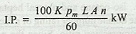# Indicated power of an IC Engine

The indicated power of an IC engine (briefly written as I.P.) is the power actually developed by the engine cylinder. Mathematically,where

K = Number of cylinders,
pm = Actual mean effective pressure in bar (1 bar = 100 kN/m2),
L = Length of stroke in meters,
A = Area of the piston in m2,
n = Number of working strokes per minute
= Speed of the engine for two stroke cycle engine
= Half the speed of the engine for four stroke cycle engine.

Note : The I.P. of a multi-cylinder of spark ignition engine is determined by Morse test.